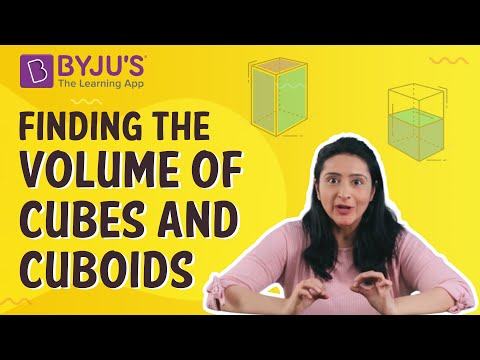# Difference between Cube and Cuboid

The key difference between a cube and a cuboid is: a cube has six square-shaped faces of the same size, but a cuboid has rectangular faces. Although both cubes and cuboids look the same in structure, they have a few different properties based on edge length, diagonals and faces.

In Geometry, many shapes such as cylinders, spheres and cones have distinct properties, but only cuboids and cubes are two such solids which have some common properties like six faces, eight vertices and twelve edges. Also, all the interior angles are equal to 90 degrees. Let us see how cubes and cuboids can be differentiated from each other.

## Definition of Cube and Cuboid

Cube: A three-dimensional shape which has six square-shaped faces of equal size and has an angle of 90 degrees between them is called a cube. It has 6 faces, 12 edges and 8 vertices. Opposite edges are equal and parallel. Each vertex meets three faces and three edges.

Cuboid: A three-dimensional figure with three pairs of rectangular faces attached opposite each other. These opposite faces are the same. Out of these six faces, two can be squares. The other names for cuboids are rectangular boxes, rectangular parallelepipeds, and right prisms.

## What are the Differences Between Cube and a Cuboid?

 S.No Topics Cube Cuboid 1 Sides All the side lengths of a cube are of equal measure. All the side lengths of a cuboid are of different measure. 2 Shape A cube is a three-dimensional form of a square. A cuboid is a three-dimensional form of a rectangle. 3 Faces All six faces of a cube are squares. All six faces of a cuboid are rectangles. 4 Diagonals The 12 diagonals on the cube surface are of the same measure. A cuboid has 12 face diagonals and 4 body/space diagonals. 5 Internal Diagonals The total of  4 internal diagonals should have the same measure. A cuboid also has 4 internal diagonals. Among that, the two pairs of internal angles are of different measures. 6 Example Rubik’s Cube, Dice Ice cube Duster, Bricks 7 LSA (Lateral Surface Area) Formula 4 × (Side) 2 2 (length + breadth) × height 8 TSA (Total Surface Area) Formula 6 × (side) 2 2 [(length × breadth) + (breadth × height) + (height × length)] 9 Diagonal Formula √3 × (side) √(length2 + breadth2 + height2) 10 Volume Formula (Side)3 length × breadth × height 11 Perimeter Formula 12 × (Side) 4 × (length + breadth + height)

## Cube and Cuboid Similarities

• A cube and cuboid have six faces.
• They both have 12 edges.
• Cube and cuboid have eight vertices.

#### For More Information On Volumes of Cubes and Cuboid, Watch The Below Video:### Solved Examples

Example 1: Determine the total surface area of cuboid that has length = 2 cm, breadth = 3 cm and height = 7 cm.

Solution: Total Surface Area(TSA) of cuboid = 2 (lb + bh + hl )

TSA = 2 ( 2×3 + 3×7 + 7×2)

TSA = 2 ( 6 + 21 + 14 )

TSA = 82

Therefore, the total surface area of this cuboid is 82 sq.cm.

Example 2: Calculate the surface area of a cube with edge length equal to 8 cm.

Solution: Given length, ‘a’= 8 cm

Surface area = 6a2

= 6× 82 = 6 ×64

= 384 cm2

Register at BYJU’S – The Learning App to know the difference between other shapes in mathematics.

## Frequently Asked Questions on the Difference Between Cube and Cuboid

Q1

### What is the key difference between cube and cuboid?

The major difference is the shape of faces of cuboid and cube. A cuboid has rectangular-shaped face and a cube has square-shaped face.
Q2

### Are cubes also called cuboid?

Cubes are also a type of cuboid which has its faces in square shape.
Q3

### Are cubes and square of same shape?

Cubes are three-dimensional figures which has six square-shaped faces whereas square is a two-dimensional shape which has all its sides equal in length.
Q4

### What is the shape of the cuboid?

A cuboid is a three-dimensional shape which has six rectangular-shaped faces, eight vertices and 12 edges. The three dimensions of cuboid are length, breadth and height.
Quiz on Cube Vs Cuboid